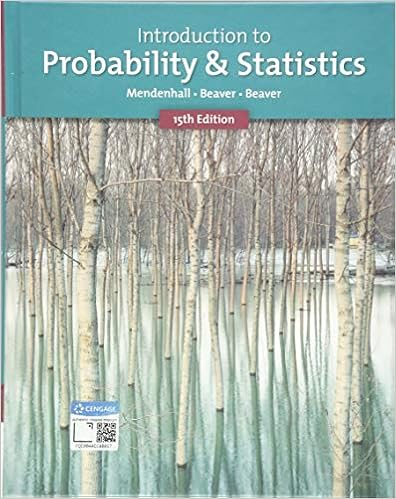# Stats 2035 - Midterm 1 - Practice 2 - Statistics...

• 4
• 100% (2) 2 out of 2 people found this document helpful

This preview shows page 1 - 2 out of 4 pages.

Stats 2035 - Midterm 1 - Practice 2

View Stats 2035 - Midterm 1 - Practice 2 from STATISTICS 2035 at Western University.

1 .   A manager has just received the expense cheques for twelve of her employees . She randomly distributes the checks to all the employees . What is the probability that exactly eleven of them will receive the correct cheques ( cheques with the correct names ) .
A ) 1
B ) 1/11
C ) 1/12
D ) 0
E ) 1/6
2 .   Consider the following sample data : 4 , 2 , 5 , 7 , 1 , 8 , 4 , 9 , 2 , 10 , 3 , 15 , 21 , 24 , 16 , 11 , 21 What is the IQR ( Interquartile Range ) of this data set ?
3 .   What is the probability that a randomly selected cup will not overflow when filled by this machine ?
5 .   You are given the following sample data ( with one data value missing ) : 6 , 1 , 4 , 2 , ___. If the mean of the sample is 3.4 , what is the missing data value ?
6 .   Let the random variable X represent the number of heads obtained when flipping a fair coin 30 times . What is the variance of X ?
A ) 15
B ) 7.5
C ) 2.74
D ) 0.25
E ) 1
7 .   Let the random variable X represent the amount of time spent studying for this exam . Suppose that X is approximately Normal with a mean , μ of 20 hours and a standard deviation , σ = 6 . The probability that a student will study for 26 or more hours is :
8 .   Suppose that students arrive at a local coffee shop at a rate of 6 per hour . What is the chance that at least one student will arrive in a half hour ?
9 .   Five coins are flipped at the same time and they all land on a table . What is the probability of obtaining at least 3 heads given that at least one tail is flipped ?
10 .   If events A and B are independent , each with a probability of 0.3 , which of the following are correct ?
A ) P( A ∩
B ) ≠ 0
B ) P( A ∪
B ) = 0.51
C ) ( ) P A B ∩ = 0.49
D ) All of the above are correct
P A ∪ B = 0.51
Answer:     P A ∪ B = 0.51
11 .   If the random variable of X is normally distributed with a mean of 50 and a standard deviation of 10 , approximately what % of all possible observed values of X will lie between 30 and 70 .
##### We have textbook solutions for you!
The document you are viewing contains questions related to this textbook.The document you are viewing contains questions related to this textbook.
Chapter 6 / Exercise 17
Introduction to Probability and Statistics
Beaver/MendenhallExpert Verified
Statistics 2035—Midterm #1 -- Practice Questions #2 1. A manager has just received the expense cheques for twelve of her employees. She randomly distributes the checks to all the employees. What is the probability that exactlyeleven of them will receive the correct cheques (cheques with the correct names). A) 1 B) 1/11 C) 1/12 D) 0 E) 1/6
2. Consider the following sample data: 4, 2, 5, 7, 1, 8, 4, 9, 2, 10, 3, 15, 21, 24, 16, 11, 21 What is the IQR (Interquartile Range) of this data set?
E) 0.5 Use the following information for the next TWO questions:An automatic dispensing machine dispenses a certain beverage into a 20 ounce cup. Let xbe the amount dispensed by the machine where xis Normally distributed with a mean of 19.8 and a standard deviation of 0.8. 3. What is the probability that a randomly selected cup will not overflow when filled by this machine? Answer: ___________ 4. It turns out that 25% of all cups filled by this machine have c-ounces or less in them. What is the value of c? Answer: ___________ 5. You are given the following sample data (with one data value missing): 6, 1, 4, 2, ___. If the mean of the sample is 3.4, what is the missing data value?
D) 4
##### We have textbook solutions for you!
The document you are viewing contains questions related to this textbook.The document you are viewing contains questions related to this textbook.
Chapter 6 / Exercise 17
Introduction to Probability and Statistics
Beaver/MendenhallExpert Verified
•••